# Multidimensional Arrays in CPP

### What is an array?

• An array is a collection of similar data items stored in a contiguous memory locations
• Here, Multidimensional Array means data in an array is stored in the tabular form  ( array of arrays )

### Syntax:

```data_type  name_of_array[size1][size2][size3]......[sizeN]
Where,
Data_type - Type of data to be stored in an array . E.g : int, double, char, etc
Name_of_array - name provided to the array
size1,size2..sizeN - Dimensions
```

Example:

```Two dimensional array,
int arr

Three dimensional array,
char  alpha
```

### Size of Multidimensional Array:

• The total number of elements present in the multi-dimensional array is the product of all dimensions
• In the above example, the number of elements present in arr is 600 ( 20*30 = 600 )
• char alpha has 6000 elements ( 10*20*30 = 6000 )

### Two Dimensional Array ( 2D Array ) :

Syntax:

```data_type name_of_array[size1][size2]
```

Example:

```int arr - This denotes that name of the array is arr and it has 2 rows and 3 columns
```

Elements in the 2D array are accessed in the form of arr[i][j] where i is the row number and j is the column number. The rows are numbered from 0 to x-1 where x is the number of rows in the array. The columns are numbered from 0 to y-1 where y is the number of columns in an array.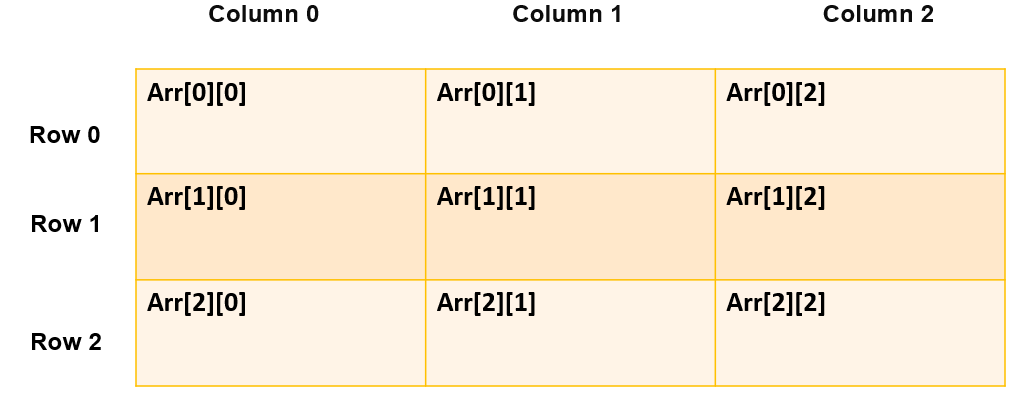### Initialization of 2D arrays:

• Type-1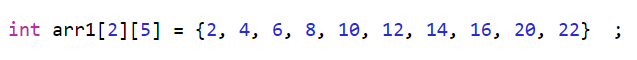The above array has 2 rows and 5 columns. The elements will be filled in the 2D array in the order mentioned above. The first 5 elements (2,4,6,8,10) will be present in the 1st row, the second 5 elements (12,14,16,20,22)  will be present in the 2nd row

• Type-2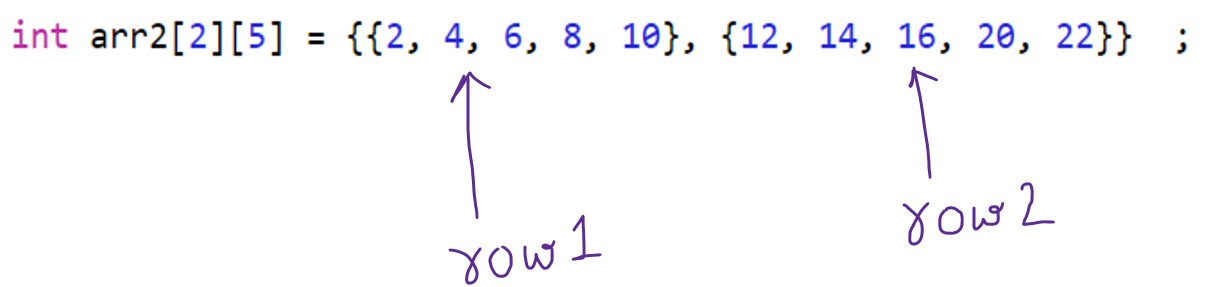Using braces is the better method compared to type-1. Each nested brace represents the Row of Array.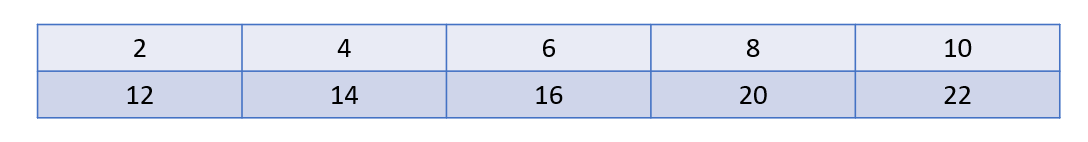Code:

## C++ Code

``````#include<bits/stdc++.h>

using namespace std;

int main() {
int array = {{1,2,3},{4,5,6}};

for (int i = 0; i < 2; i++) {
for (int j = 0; j < 3; j++) {

cout << " array[" << i << "][" << j << "] = " << array[i][j] << endl;

}
}
return 0;
}``````

Output:

array = 1
array = 2
array = 3
array = 4
array = 5
array = 6

### Three Dimensional Array ( 3D Array )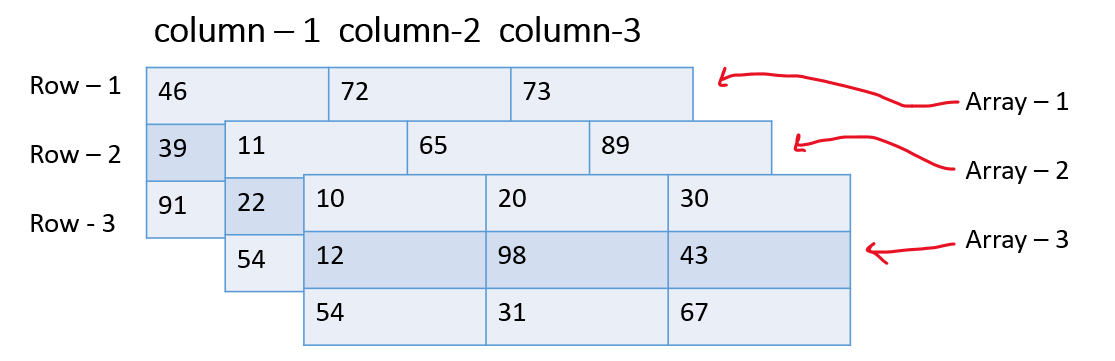### Initialization of 3D arrays:

Initialization is the same compared to the 2D array only difference is we need to add 1 extra dimension.

• Type 1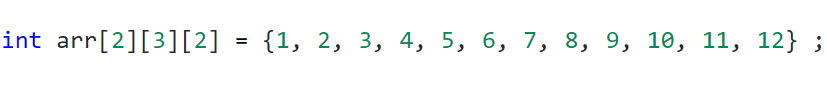• Type 2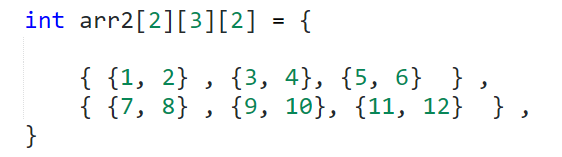Accessing elements in a 3d array is similar to a 2d array. The only difference is we add an extra dimension to it.

Code:

## C++ Code

``````#include<bits/stdc++.h>

using namespace std;

int main() {
int array = {
{ {11, 12}, {13, 14}, {15, 16} },
{ {17, 18}, {19, 20}, {21, 22} }
};

for (int i = 0; i < 2; i++) {
for (int j = 0; j < 3; j++) {
for (int k = 0; k < 2; k++) {
cout << " array[" << i << "][" << j << "][" << k << "] = " <<
array[i][j][k] << endl;
}
}
}
return 0;
}``````

Output:

array = 11
array = 12
array = 13
array = 14
array = 15
array = 16
array = 17
array = 18
array = 19
array = 20
array = 21
array = 22

Special thanks to Shreyas Vishwakarma for contributing to this article on takeUforward. If you also wish to share your knowledge with the takeUforward fam, please check out this article